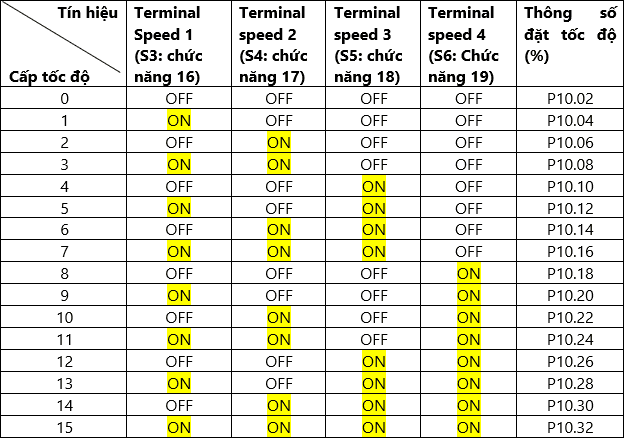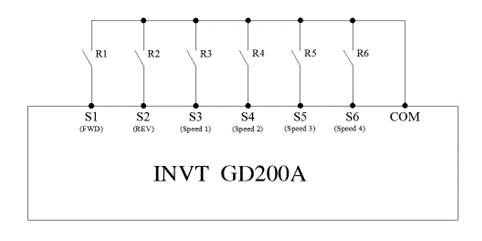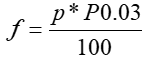02.03.2023

# Guide to Setting Up Multi-Speed VFD

VFDs come with various functions to adjust speed, and users often want to understand how to set up a multi-speed VFD.

The multi-speed function of VFDs is widely applied in the industry. Depending on the technological requirements of the machinery, users can control the input signals to set the speed levels accordingly. This article provides a detailed guide on setting up a multi-speed VFD for easy implementation.

### 1. Overview of Multi-Speed VFD

The multi-speed mode of VFDs is applied to control the speed change requirements at different stages of technology. The motor can switch between different speed levels, depending on the system’s needs. This enhances efficiency and precision in controlling motors for industrial applications.

### 2. Steps for Setting Up Multi-Speed VFD

Each type of VFD will have different settings for multi-speed operation. However, most follow a common process:

Step 1: Determine the wiring diagram and multi-speed terminals.

Step 2: Set up the multi-speed function.

Step 3: Set the desired frequency when activating the multi-speed function.

### 3. Instructions for Setting Up INVT VFD for Multi-Speed Operation

The INVT VFD can control 16 speed levels for the motor. By using a combination of 4 input signals, the VFD can control the motor’s speed levels as needed.

#### 3.1 Control Signal Table for Speed Levels#### 3.2 Connection Diagram

INVT VFD Controls Motor in Forward and Reverse, with 16 Speed Levels (Using 4 ON/OFF Input Signals).In the diagram, R1, R2, R3, R4, R5, R6 are the contacts of intermediate relays, PLC outputs, control boards, etc. When R1 is ON, the VFD runs forward; when R2 is ON, the VFD runs in reverse. When R3, R4, R5, R6 are ON or OFF, they correspond to the speed levels in Table 1.

#### 3.3 Setting Parameters

P00.01 = 1                 Select RUN/STOP from the terminal

P00.06 = 6                Select the source for setting speed using the multi-speed control channel

P05.01 = 1                  Select S1 Function: Forward rotation.

P05.02 = 2                 Select S2 Function: Reverse rotation.

P05.03 = 16               Select S3 Function: Multi-speed control signal 1

P05.04 = 17               Select S4 Function: Multi-speed control signal 2.

P05.05 = 18               Select S5 Function: Multi-speed control signal 3.

P05.06 = 19               Select S6 Function: Multi-speed control signal 4.

P10.02 = …%             Speed Level 0 Percentage Setting

P10.04 = …%            Speed Level 1 Percentage Setting

P10.06 = …%            Speed Level 2Percentage Setting

P10.08 = …%            Speed Level 3 Percentage Setting

P10.10 = …%            Speed Level 4 Percentage Setting

P10.12 = …%            Speed Level 5 Percentage Setting

P10.14 = …%            Speed Level 6 Percentage Setting

P10.16 = …%            Speed Level 7 Percentage Setting

P10.18 = …%            Speed Level 8 Percentage Setting

P10.20 = …%            Speed Level 9 Percentage Setting

P10.22 = …%            Speed Level 10 Percentage Setting

P10.24 = …%            Speed Level 11 Percentage Setting

P10.26 = …%            Speed Level 12 Percentage Setting

P10.28 = …%            Speed Level 13 Percentage Setting

P10.30 = …%            Speed Level 14 Percentage Setting

P10.32 = …%            Speed Level 15 Percentage Setting

Note:

• A positive percentage results in forward rotation when a run command is issued to the VFD. A negative percentage leads to reverse rotation when a run command is given to the VFD.
• The percentage corresponds to the ratio with the Max Frequency (P00.03).Trong đó:

• f: represents the desired frequency for the set speed level
• p: stands for the percentage set for the speed level
• 03: corresponds to the maximum frequency parameter of the VFD

For any questions or additional information, please contact the Hotline at 1800 6567 to receive prompt support from DAT Group – the authorized representative of INVT in Vietnam.